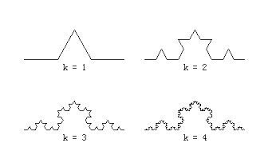# 重修微积分7——测度精选

$m(\phi )=0,\;\;\; m(\bigcup_{n=1}^\infty E_n)=\sum _{n=1}^{\infty} m(E_n),\;\; E_i \cap E_j =\phi\;\;\forall i\neq j$

$\mathbb{R}^n$空间中的一个点的集合，可以包含在任意小的区间里，它的勒贝格测度小于任何正数，所以它只能为0。从测度的定义可知，可数个零测集的并集仍然是零测集，所以有理数集合是实数空间$\mathbb{R}$上的零测集。

n维欧几里德空间，任何集合A都可以被一族可数的开集覆盖，这族开覆盖测度和的下确界称为集合A的外测度，记为m*(A)。外测度对所有集合都有定义，保持有测度的非负性，对集合包含关系的单调性，和次可数可加性。当集合A是可测时，外测度等于它的测度。

$m^*(A) \ge 0, \; m^*(\phi)=0,\;\;\; A\subset B \Rightarrow m^*(A)\le m^*(B),$

$m^*( \bigcup _{n=1}^\infty E_n) \le \sum _{n=1}^\infty m^*(E_n),\;\; E_i\cap E_j = \phi\;\;\forall i\neq j$

Koch曲线是这样构造的。对单位线段，中间1/3用等边三角形的两边来代替，得到四条边的曲线k=1，对这四条边做同样的替换，得到k=2曲线，如此无限重复这个替代过程，它趋向Koch曲线。（见图，图像抄自网络）$H_d^s(A)=\inf \left\{\sum_{i=1}^\infty |O_i|^s \mid \bigcup_{i=1}^\infty O_i \supset A, \;\; |O_i| \le d\right \}$$H^s(A)=\lim_{d\rightarrow 0}H_d^s(A)$

Hs(A)定义在$\mathbb{R}^n$Borel集上，不难验证它满足可数可加性，所以是个带参数s的测度。当s=n时，Hs(A)$\mathbb{R}^n$n维勒贝格测度（精确地说只差一个与n有关的倍因子，因为Hausdorff测量的尺子是球，勒贝格是方块）。

$\mathbb{R}^n$空间，将几何体A线性放大k倍，其集合记为$kA =\{kx \; | \; x\in A\}$，则有 $H^s(kA)=k^sH^s(A)$，这与k维几何体的线性放大后，长度、面积和体积比例关系是一致的。注意到对于给定的集合AHausdorff测度Hs(A)，随着sn+1开始减小，其数值从0，到了一个临界点后，突然跳到无穷大，我们把这个s的临界值，称为几何体的维数，或者Hausdorff维数。当它是自然数时，这与我们日常中的经验是一致的，但有时它不是一个整数。

（待续）

【扩展阅读】

2. WikipediaHausdorffmeasure http://en.wikipedia.org/wiki/Hausdorff_measure

https://blog.sciencenet.cn/blog-826653-888365.html

## 全部精选博文导读

GMT+8, 2023-9-26 06:00